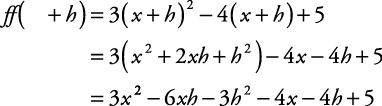## Polynomial Function

A polynomial function is any function of the form

P( x) = a 0 x n + a 1 x n – 1 + a 2 x n – 2 + ... + a n – 1 x + a n

where the coefficients a 0, a 1, a 2, ..., a n are real numbers and n is a whole number. Polynomial functions are evaluated by replacing the variable with a value. The instruction “evaluate the polynomial function P( x) when x is replaced with 4” is written as “find P(4).”

##### Example 1

If P( x) = 3 x 3 – 2 x 2 + 5 x + 3, find P(–4).##### Example 2

If f ( x) = 3 x 2 – 4 x + 5, find f ( x + h).Back to Top
A18ACD436D5A3997E3DA2573E3FD792A